# Using Strategy Using Strategy Scan Path Strategy It

• Slides: 20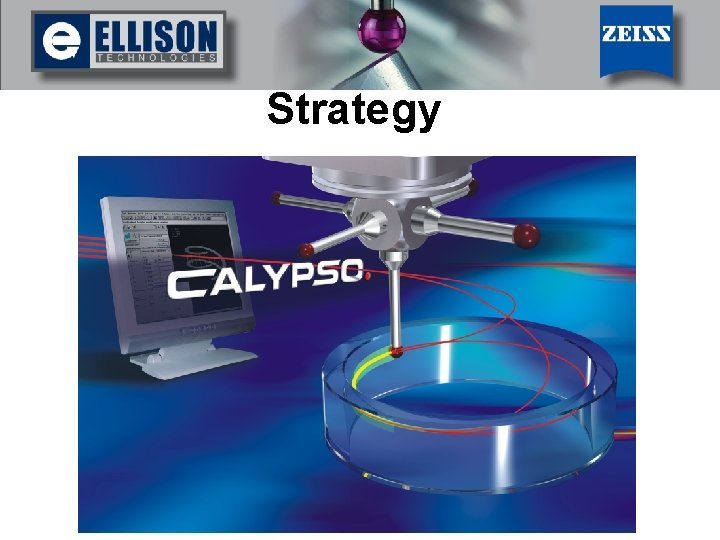Using Strategy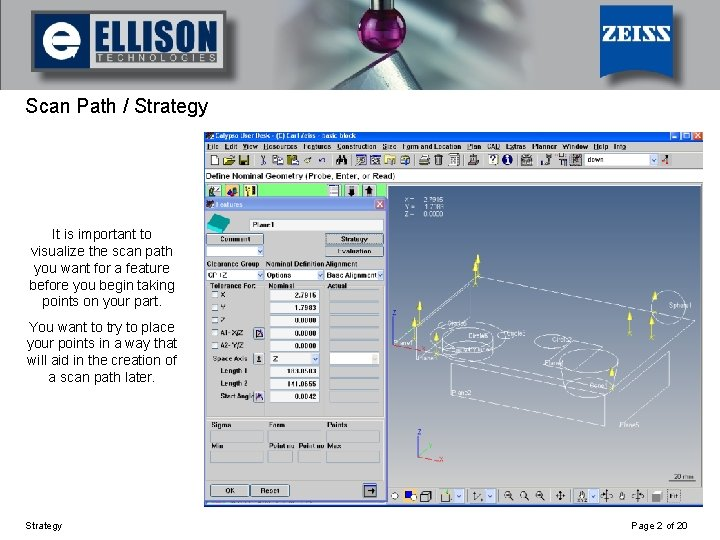Using Strategy Scan Path / Strategy It is important to visualize the scan path you want for a feature before you begin taking points on your part. You want to try to place your points in a way that will aid in the creation of a scan path later. Strategy Page 2 of 20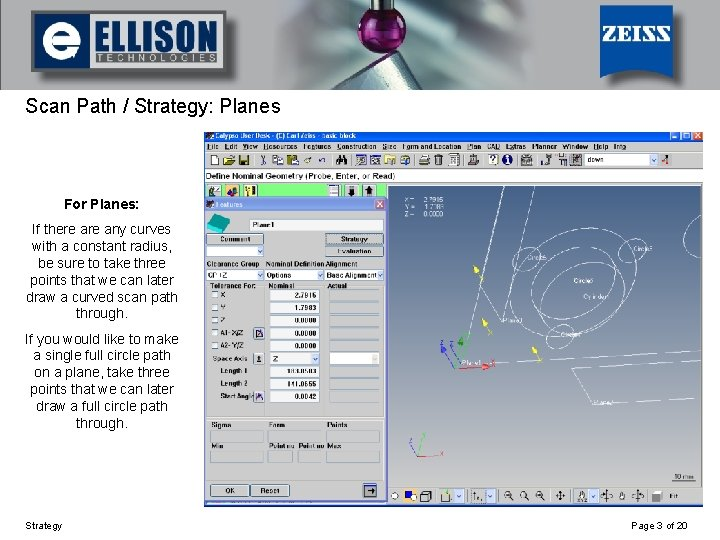Using Strategy Scan Path / Strategy: Planes For Planes: If there any curves with a constant radius, be sure to take three points that we can later draw a curved scan path through. If you would like to make a single full circle path on a plane, take three points that we can later draw a full circle path through. Strategy Page 3 of 20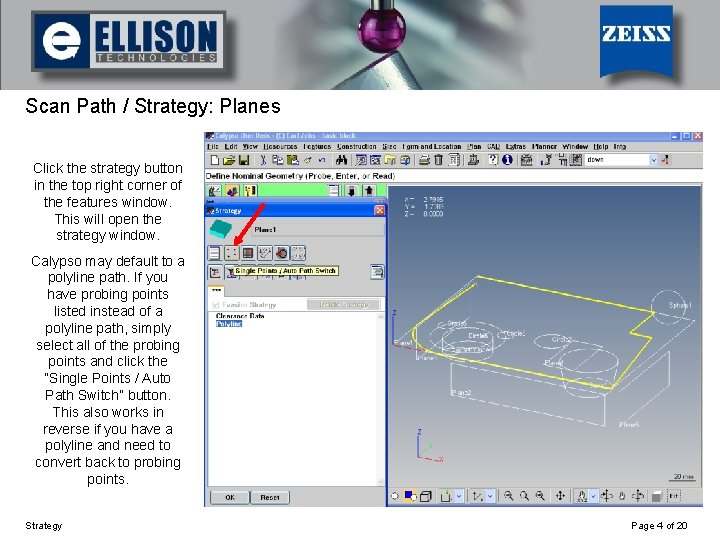Using Strategy Scan Path / Strategy: Planes Click the strategy button in the top right corner of the features window. This will open the strategy window. Calypso may default to a polyline path. If you have probing points listed instead of a polyline path, simply select all of the probing points and click the “Single Points / Auto Path Switch” button. This also works in reverse if you have a polyline and need to convert back to probing points. Strategy Page 4 of 20Using Strategy Scan Path / Strategy: Planes Double click the polyline to open the polyline window. Here you can make several changes to the scan path including speed, step width, number of points, and changing segments of the path from straight lines to arc or circle paths. Strategy Page 5 of 20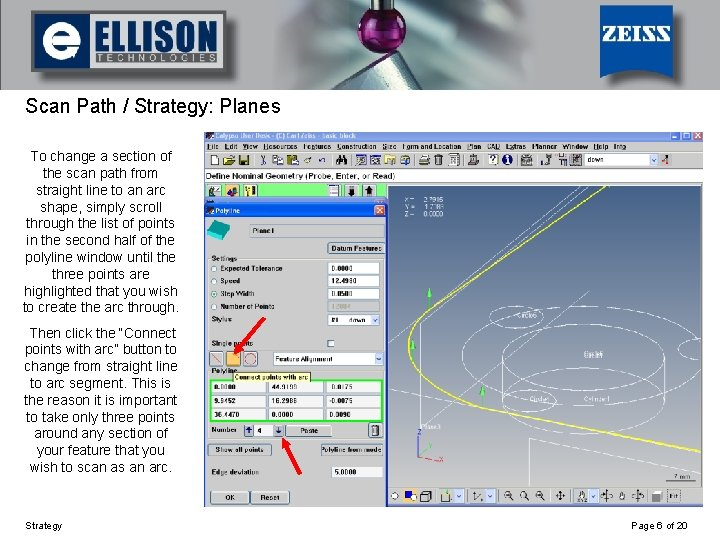Using Strategy Scan Path / Strategy: Planes To change a section of the scan path from straight line to an arc shape, simply scroll through the list of points in the second half of the polyline window until the three points are highlighted that you wish to create the arc through. Then click the “Connect points with arc” button to change from straight line to arc segment. This is the reason it is important to take only three points around any section of your feature that you wish to scan as an arc. Strategy Page 6 of 20Using Strategy Scan Path / Strategy: Planes Here we see that a circular scan path is used on a plane to measure around a cone shaped hole in the part. This plane was created by probing only three points around the perimeter of the hole. The strategy was then changed into a circular path by opening the Polyline window and clicking the “Connect points with complete circle” button. The result is a uniform circular scan path located on the plane. Strategy Page 7 of 20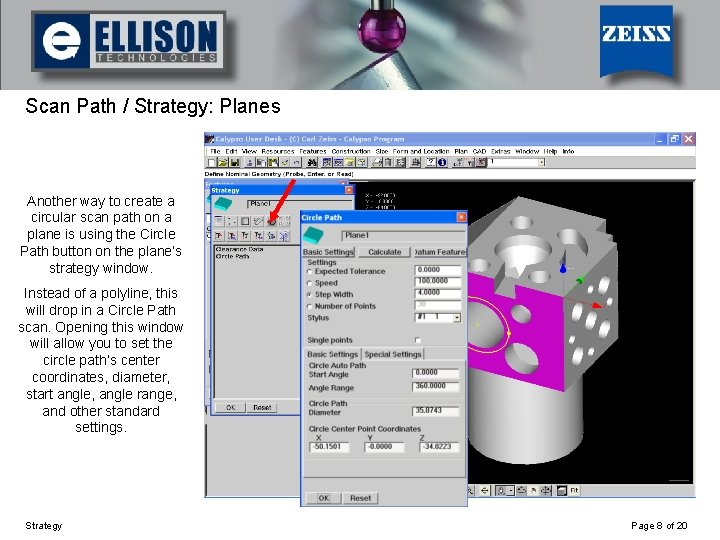Using Strategy Scan Path / Strategy: Planes Another way to create a circular scan path on a plane is using the Circle Path button on the plane’s strategy window. Instead of a polyline, this will drop in a Circle Path scan. Opening this window will allow you to set the circle path’s center coordinates, diameter, start angle, angle range, and other standard settings. Strategy Page 8 of 20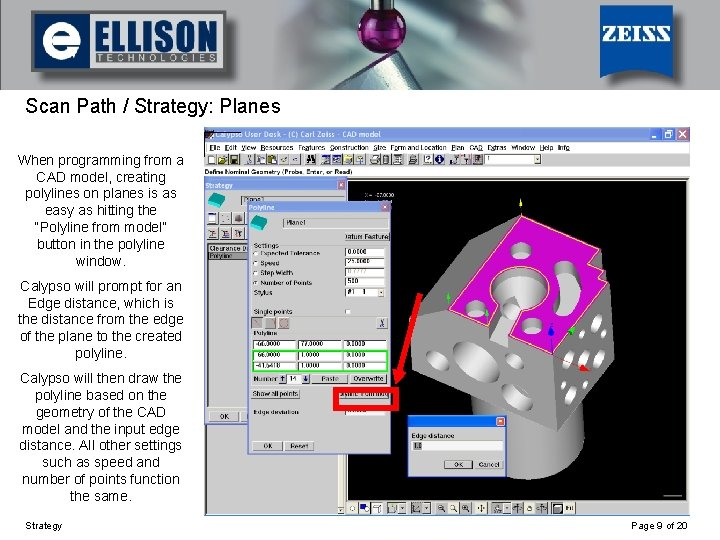Using Strategy Scan Path / Strategy: Planes When programming from a CAD model, creating polylines on planes is as easy as hitting the “Polyline from model” button in the polyline window. Calypso will prompt for an Edge distance, which is the distance from the edge of the plane to the created polyline. Calypso will then draw the polyline based on the geometry of the CAD model and the input edge distance. All other settings such as speed and number of points function the same. Strategy Page 9 of 20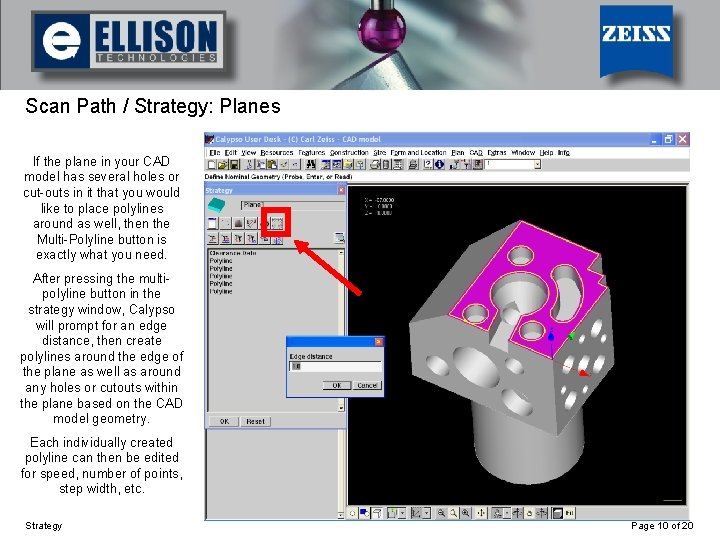Using Strategy Scan Path / Strategy: Planes If the plane in your CAD model has several holes or cut-outs in it that you would like to place polylines around as well, then the Multi-Polyline button is exactly what you need. After pressing the multipolyline button in the strategy window, Calypso will prompt for an edge distance, then create polylines around the edge of the plane as well as around any holes or cutouts within the plane based on the CAD model geometry. Each individually created polyline can then be edited for speed, number of points, step width, etc. Strategy Page 10 of 20Using Strategy Scan Path / Strategy: Circles This is what the strategy window looks like for a circle. A circle auto path may be automatically generated in this window. If there is no circle auto path, one can be inserted by clicking the “Circle Auto Path Definition” button in this window. Strategy Page 11 of 20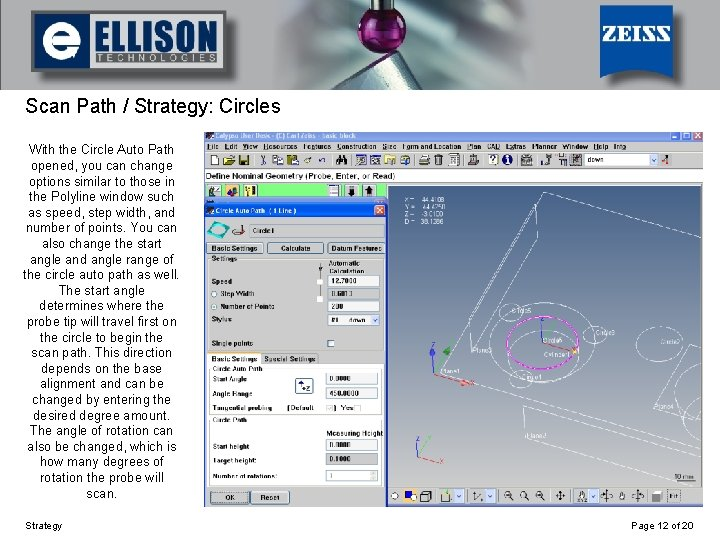Using Strategy Scan Path / Strategy: Circles With the Circle Auto Path opened, you can change options similar to those in the Polyline window such as speed, step width, and number of points. You can also change the start angle and angle range of the circle auto path as well. The start angle determines where the probe tip will travel first on the circle to begin the scan path. This direction depends on the base alignment and can be changed by entering the desired degree amount. The angle of rotation can also be changed, which is how many degrees of rotation the probe will scan. Strategy Page 12 of 20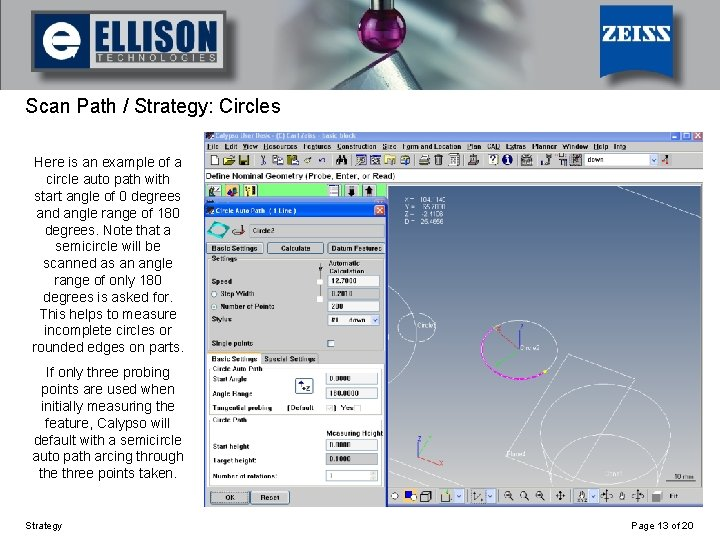Using Strategy Scan Path / Strategy: Circles Here is an example of a circle auto path with start angle of 0 degrees and angle range of 180 degrees. Note that a semicircle will be scanned as an angle range of only 180 degrees is asked for. This helps to measure incomplete circles or rounded edges on parts. If only three probing points are used when initially measuring the feature, Calypso will default with a semicircle auto path arcing through the three points taken. Strategy Page 13 of 20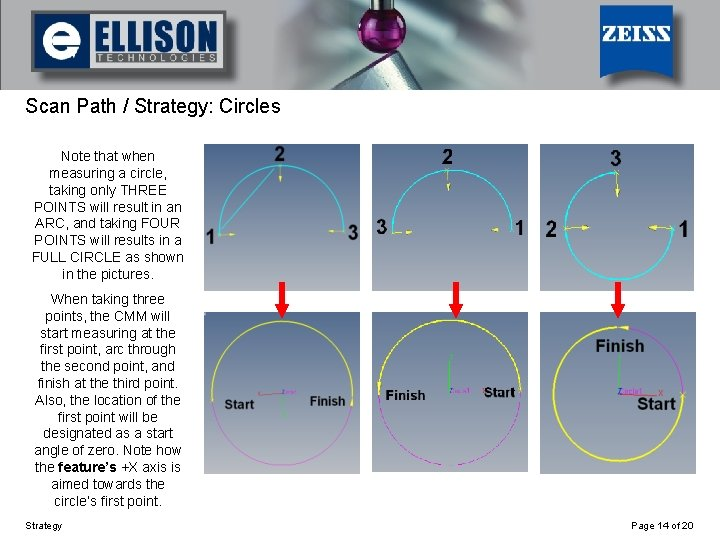Using Strategy Scan Path / Strategy: Circles Note that when measuring a circle, taking only THREE POINTS will result in an ARC, and taking FOUR POINTS will results in a FULL CIRCLE as shown in the pictures. When taking three points, the CMM will start measuring at the first point, arc through the second point, and finish at the third point. Also, the location of the first point will be designated as a start angle of zero. Note how the feature’s +X axis is aimed towards the circle’s first point. Strategy Page 14 of 20Using Strategy Scan Path / Strategy: Circles If the circle is measured by taking four or more points, the CMM will measure a full 360 degree circle. Also, Calypso will set a start angle of zero to the right side of the circle (similar to a unit circle). Note that it doesn’t matter what order we take the points in, the end result is the same: a full circle with a start angle of zero to the right side of the circle. Strategy Page 15 of 20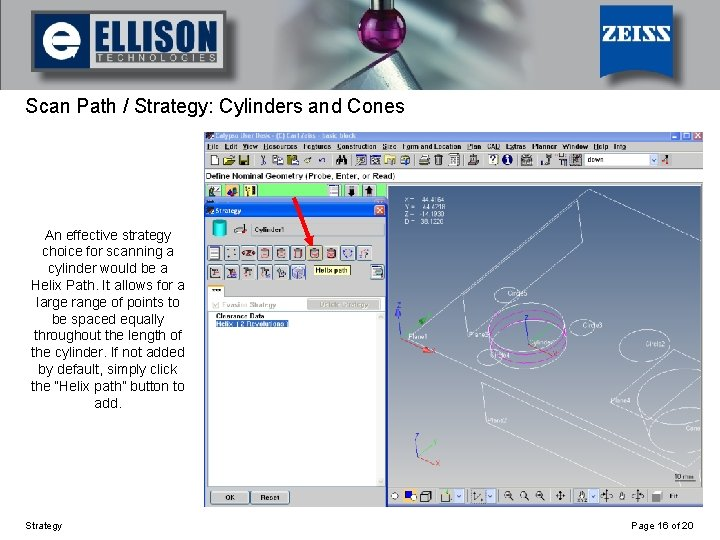Using Strategy Scan Path / Strategy: Cylinders and Cones An effective strategy choice for scanning a cylinder would be a Helix Path. It allows for a large range of points to be spaced equally throughout the length of the cylinder. If not added by default, simply click the “Helix path” button to add. Strategy Page 16 of 20Using Strategy Scan Path / Strategy: Cylinders and Cones In the Helix Path window, you can edit the start height and target height of the scan, which changes the height at which the scan will begin and end. The number of rotations and the start angle can also be edited, as well as the typical strategy options such as speed, step width, and number of points. If it is desired to scan the helix path in a Left rotation direction, simply un-select the “Right” checkbox at the bottom of this window. Strategy Page 17 of 20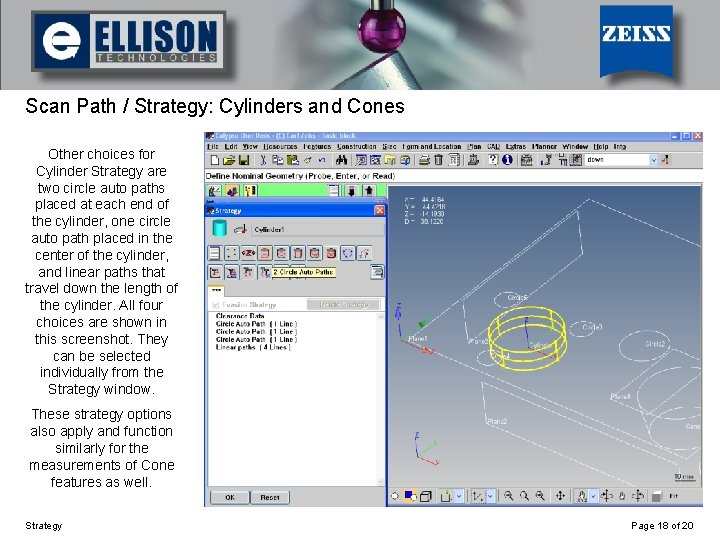Using Strategy Scan Path / Strategy: Cylinders and Cones Other choices for Cylinder Strategy are two circle auto paths placed at each end of the cylinder, one circle auto path placed in the center of the cylinder, and linear paths that travel down the length of the cylinder. All four choices are shown in this screenshot. They can be selected individually from the Strategy window. These strategy options also apply and function similarly for the measurements of Cone features as well. Strategy Page 18 of 20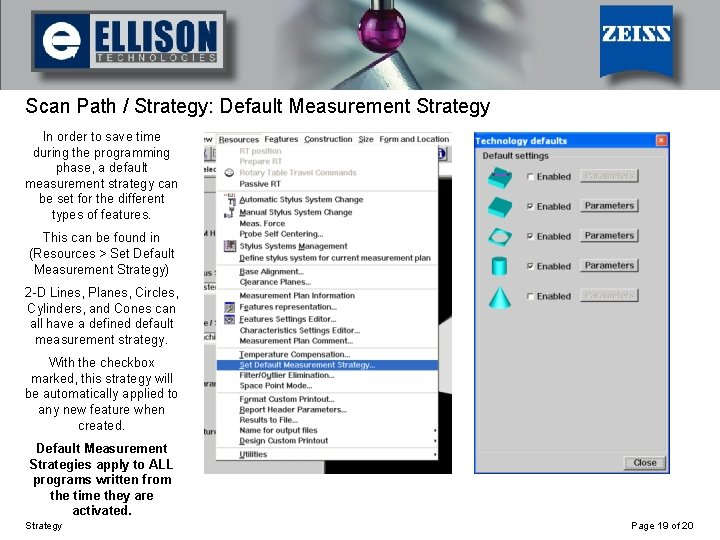Using Strategy Scan Path / Strategy: Default Measurement Strategy In order to save time during the programming phase, a default measurement strategy can be set for the different types of features. This can be found in (Resources > Set Default Measurement Strategy) 2 -D Lines, Planes, Circles, Cylinders, and Cones can all have a defined default measurement strategy. With the checkbox marked, this strategy will be automatically applied to any new feature when created. Default Measurement Strategies apply to ALL programs written from the time they are activated. Strategy Page 19 of 20Using Strategy Scan Path / Strategy: Default Measurement Strategy Click the Parameters button for the feature you wish to set a default measurement strategy for. This will bring you to a familiar Strategy window in which you can choose how the feature will be measured by default. Polylines, circle auto paths, helix paths; all are available to choose from for their respective features. Opening the selected strategy will give you more customizable options such as speed, step width, number of points, start angle, angle range, number of rotations, and so on. Strategy Page 20 of 20